# Flag

(diff) ← Older revision | Latest revision (diff) | Newer revision → (diff)
of typein an-dimensional vector spaceA collection of linear subspaces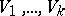ofof corresponding dimensions, such that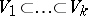(here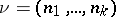,;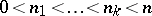). A flag of typeis called a complete flag or a full flag. Any two flags of the same type can be mapped to each other by some linear transformation of, that is, the set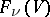of all flags of typeinis a homogeneous space of the general linear group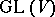. The unimodular group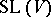also acts transitively on. Here the stationary subgroup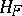ofin(and also in) is a parabolic subgroup of(respectively, of). Ifis a complete flag in, defined by subspaces, thenis a complete triangular subgroup of(respectively, of) relative to a basis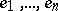ofsuch that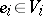,. In general, quotient spaces of linear algebraic groups by parabolic subgroups are sometimes called flag varieties. For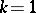, a flag of typeis simply an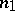-dimensional linear subspace ofandis the Grassmann manifold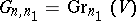. In particular,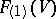is the projective space associated with the vector space. Every flag varietycan be canonically equipped with the structure of a projective algebraic variety (see ). Ifis a real or complex vector space, then all the varietiesare compact. Cellular decompositions and cohomology rings of theare known (see , and also Bruhat decomposition).